### Infinity

In mathematics, infinity isn't a vague idea, approximation, or metaphor. Infinity is just as "real" as $1,$ $99,$ and $2\pi,$ although it behaves very differently from points picked off the real number line.

In this course, you'll learn what infinity is, how it's used in mathematics, and why it's indispensable everywhere from the fundamentals of Euclidean geometry and algebra to advanced theory in calculus, discrete mathematics, and non-Euclidean geometry.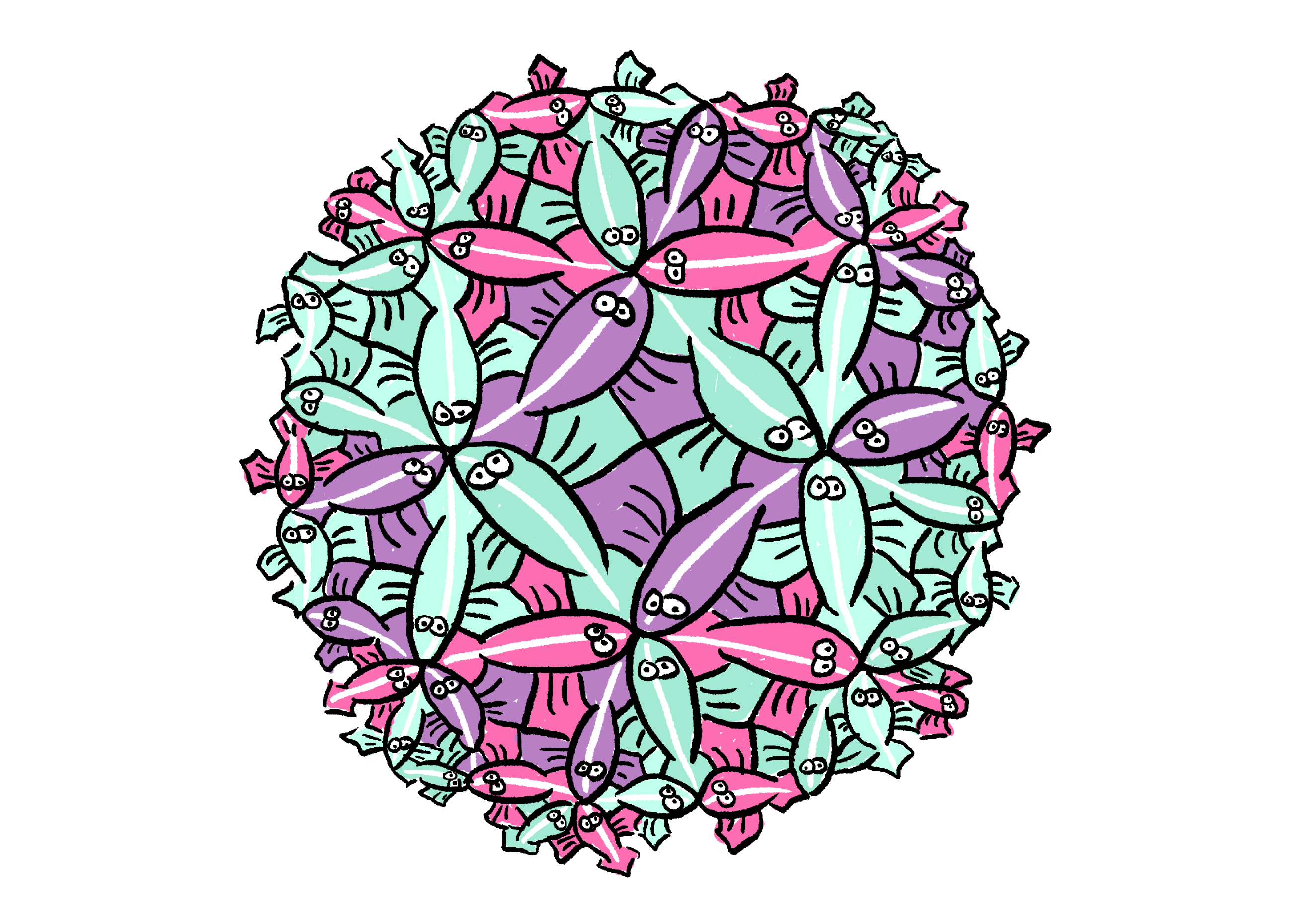"Give a man a fish and it will fill his belly for a day; give a man a hyperbolic tessellation of fish and it will fill his mind for the rest of his life." ~ Anonymous

# What's Infinite?

In this quiz, we'll start by confronting examples of the infinity inherent in algebra and Euclidean geometry. Even just figuring out "what is infinite and what is finite?" is not always straightforward.

Which of these is infinite?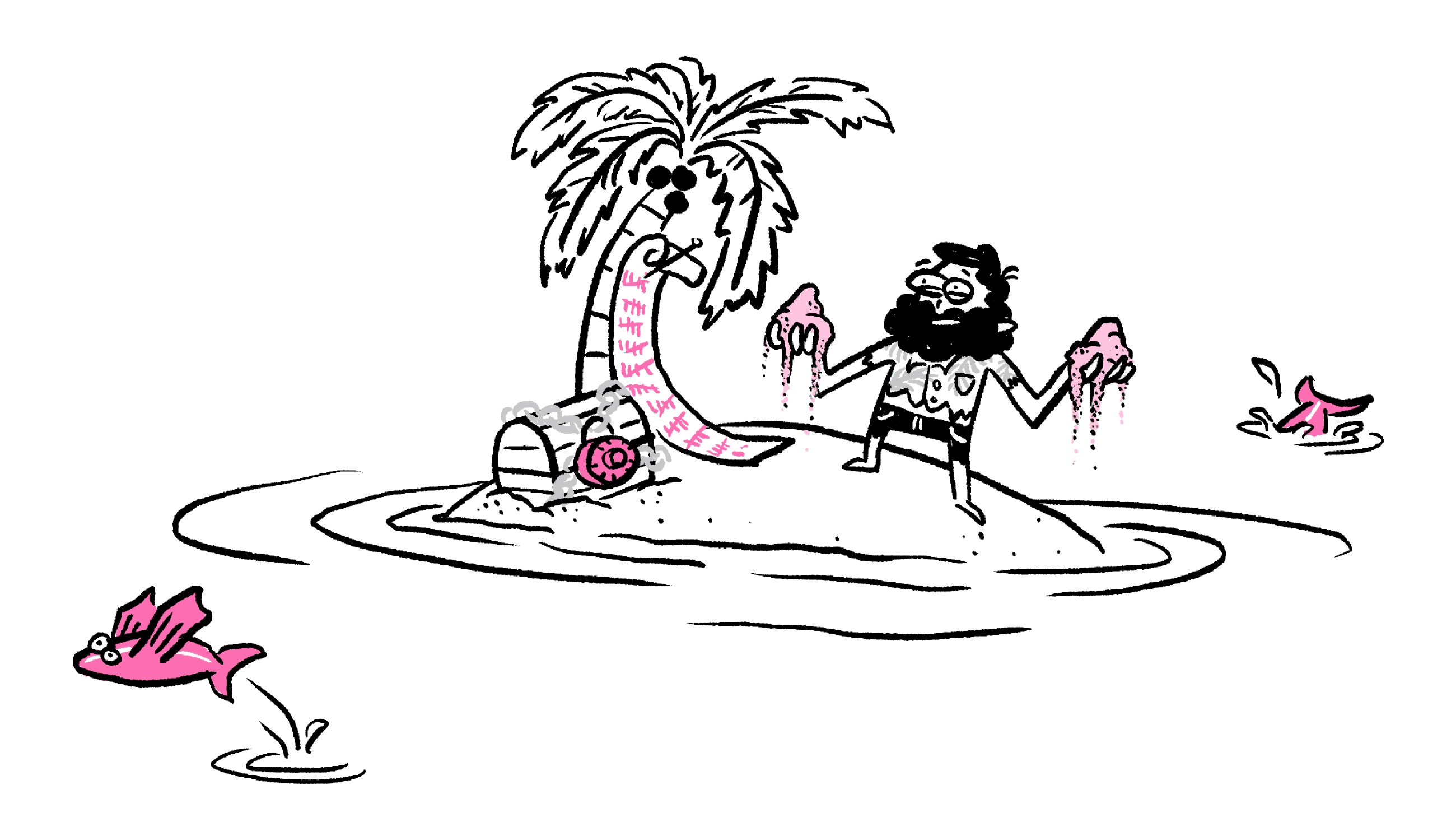Day $52$ marooned on the island: Ate fish for breakfast, lunch, and dinner. Tried another thousand lock combinations on the treasure chest, no luck yet.

# What's Infinite?

Select one or more

The major distinction between finite and infinite is a bit more slippery than just saying "there are infinitely many whole numbers because you could count them forever." Please don't try this proof technique at home, we don't have forever to spend counting...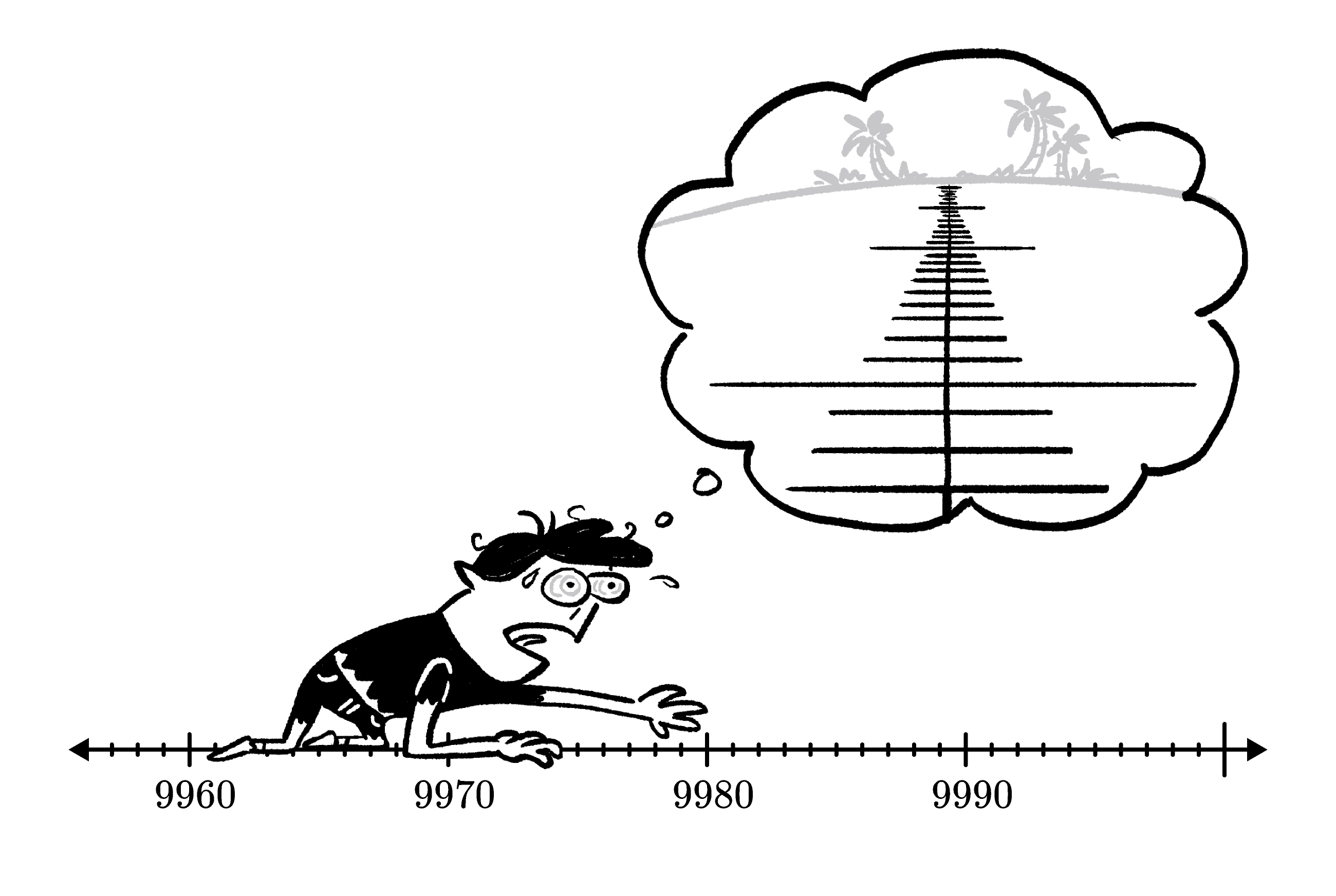# What's Infinite?

If you're asked to prove that there are infinitely many whole numbers, not even an entire life spent counting counts as a "proof." However, here's a logical argument that takes only a few minutes to say:

1. If there were finitely many whole numbers, there would be a largest whole number.
2. There is no largest whole number because, for any positive whole number $x, \text{ \_\_\_\_\_\_\_\_}$ is also a whole number and it is larger than $x.$
3. Therefore, there must be infinitely many whole numbers.$\\[-0.7em]$

Which of these options can fill the blank in the proof above?

# What's Infinite?

The continuity of lines in geometry and calculus motivates another flavor of infinity: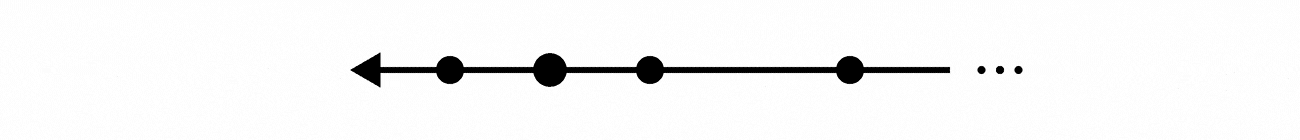On a line or line segment, any two points that have any non-zero distance between them have infinitely many points between them.

For example, there is always a point exactly halfway between any two points on a line segment. And, as we'll discuss later in this course, this also corresponds to the fact that there is always a decimal value that's exactly halfway between any pair of different decimal values.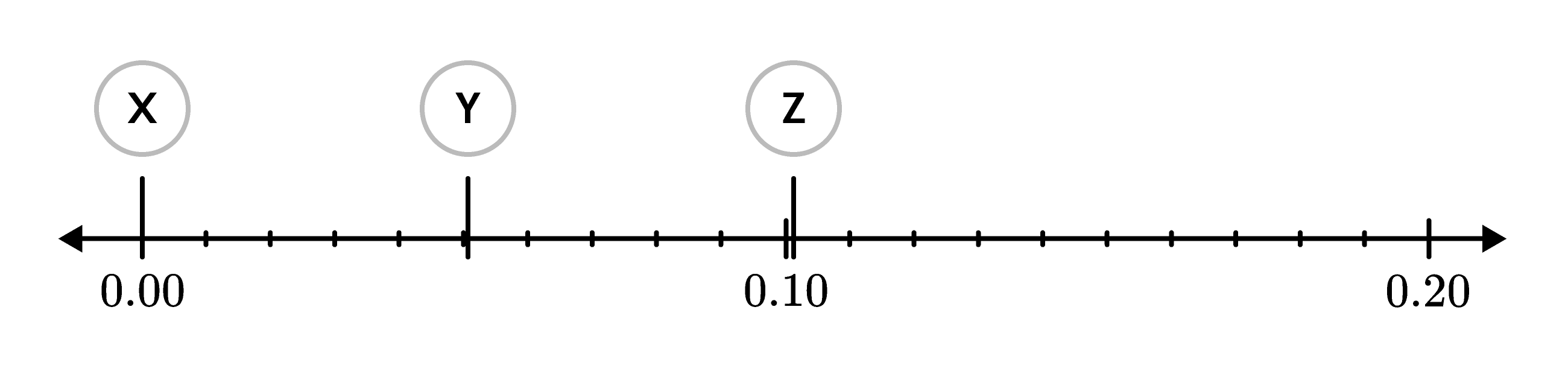If the two positions $X$ and $Z$ on the line above are a distance of $0.101$ apart and if $Y$ is exactly halfway between $X$ and $Z,$ what is the distance from $X$ to $Y?$

# What's Infinite?

Geometry has a lot of inherent infinities that are built into the nature of common, geometric objects. Even empty geometric space is an infinitude of sorts. Again, this means that figuring out "what is infinite and what is finite?" is not always straightforward.

Which of these is a finite quantity?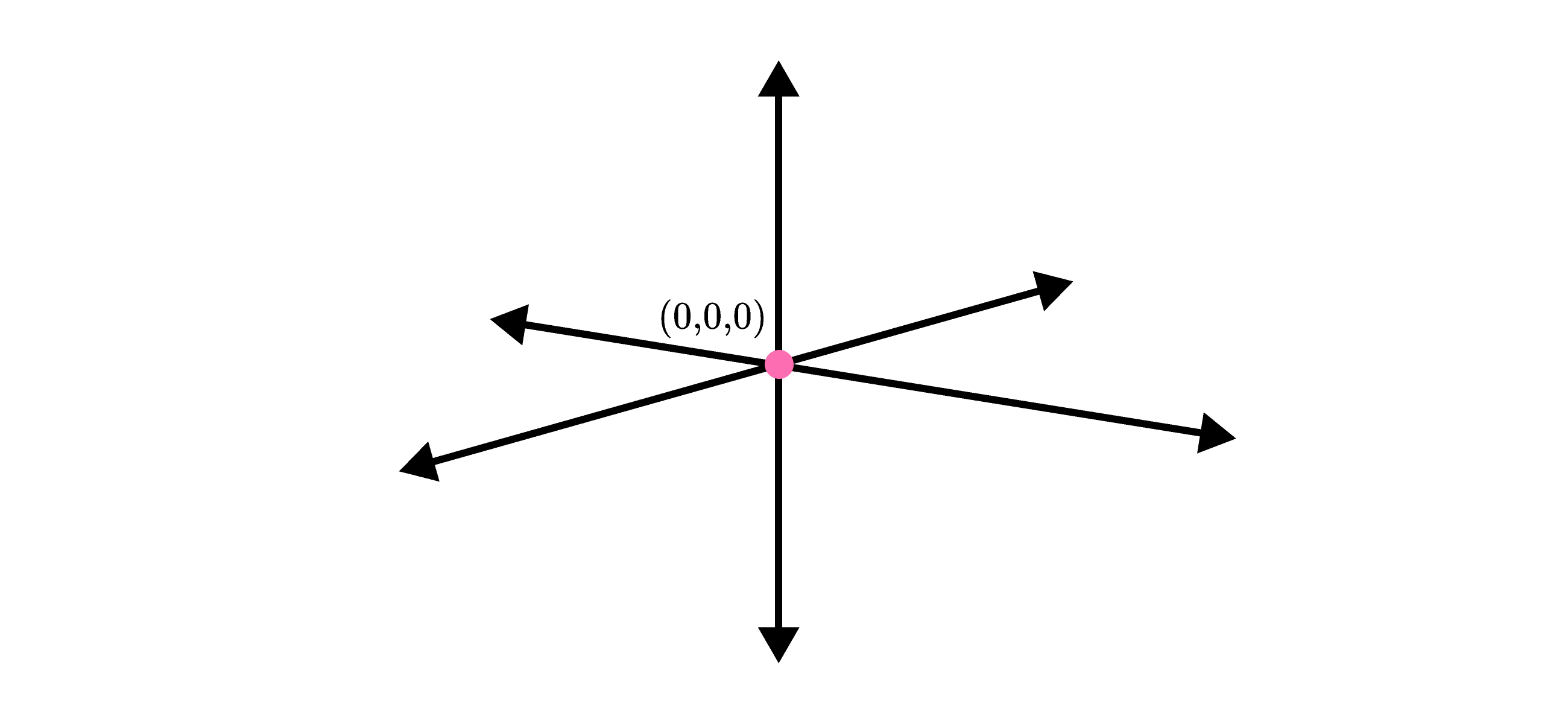# What's Infinite?

Select one or more

Is this argument about the figure below true or false?

The perimeter of this regular polygon increases every time the number of sides $(n)$ increases. Therefore, eventually, the length of the polygon's perimeter will be larger than the circumference of the gray circle surrounding it.

# What's Infinite?

In the animation above, the pink dots are marked in so that they split each line segment in the figure into thirds. Then, a small equilateral triangle is built on the middle third of each segment. And, lastly, the one old side of each new, small triangle fades away. This process is then repeated with the newly formed figure, step by step evolving the original equilateral triangle into a fractal snowflake.

If we suppose the initial triangle has an area of one square unit, is the following claim true or false?

No matter how many times the steps described above are carried out, the total area of the snowflake will never exceed two square units.

# What's Infinite?

In this quiz, you saw some examples of infinity in algebra, geometry, and a quick proof that there are infinitely many numbers. We were comparing the finite to the infinite.

In the next quiz, to really get at the essence of infinity, we need to find a way to measure and compare different infinities to each other. For example, is there any sense where the number of integers is larger than the number of positive integers, or are they, in reality, the same infinity?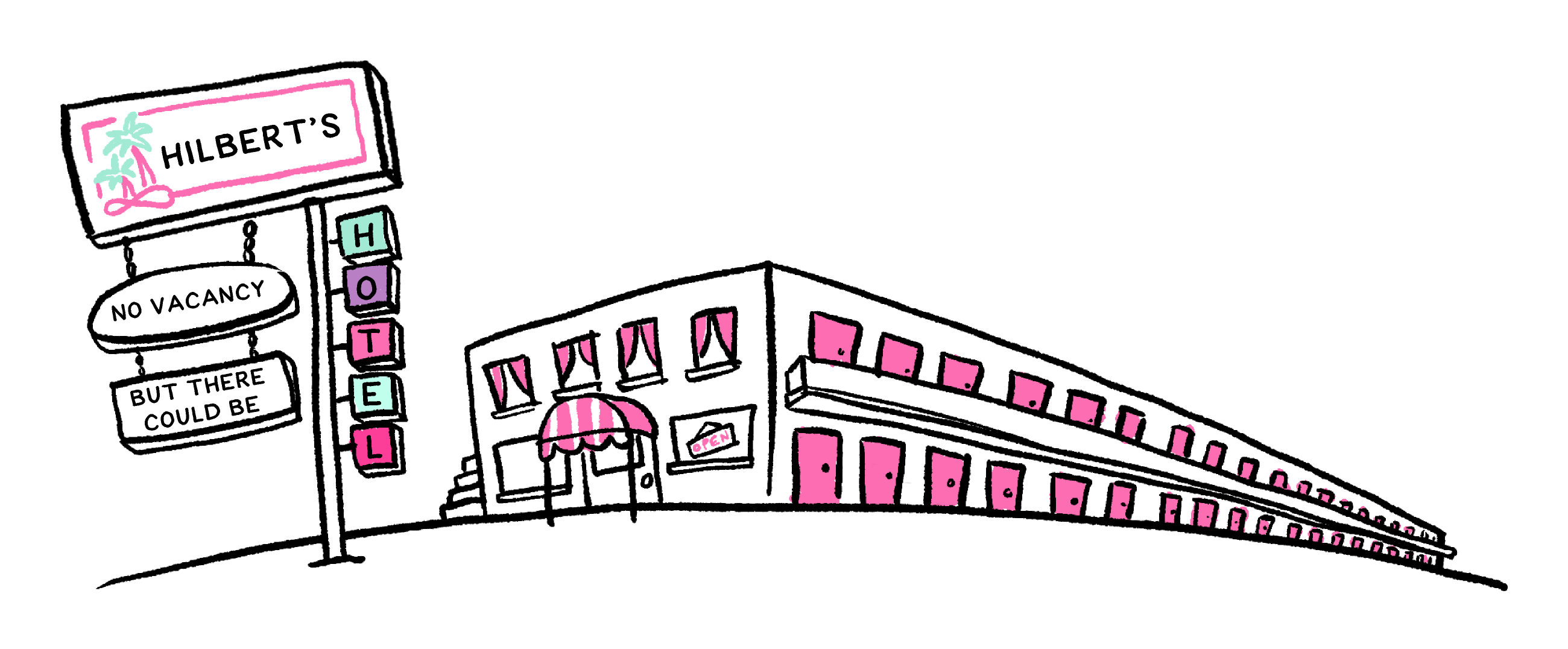# What's Infinite?

×